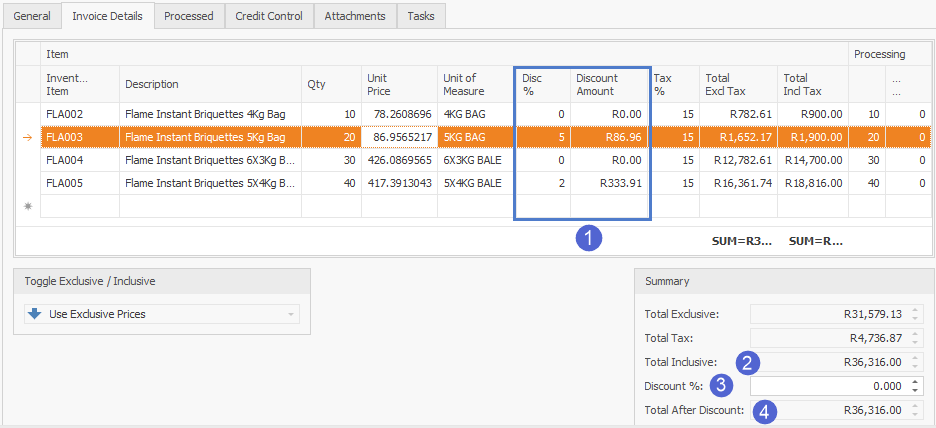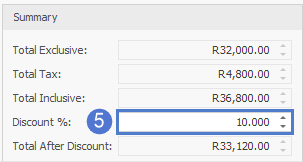# Types of Discounts that Apply to Documents

In Paradigm, there are 2 types of discounts that can be applied to a document, namely Line Discount and Document Discount.

Lines are added to a document and a discount can be applied to specific lines on that document. This is the Line Discount.

The Document Discount applies a discount to the whole document . The discounts can all apply at the same time or individually.

Line Discount

1. These columns show the discount per line.

2. This value is the total AFTER the discount is deducted per lines above.

3. This field is an additional discount option to apply to the whole document.

4. This is the total after an ADDITIONAL discount has been applied to the document.The line discount is applied to the exclusive Unit Price:

Qty*Unit Price*(Disc%/100)= Discount Amount

e.g. 20*R86.9565217*(5/100) = R86.96

Total Exclusive = Qty*Unit Price - Discount Amount

e.g. 20*R86.9565217 - R86.96 = R1652.17

Document Discount5. This option applies the discount to the whole document.

The document discount is applied to the Total Inclusive:

Total Inclusive - [Total Inclusive*(Discount%/100)]= Total After Discount

e.g. R36800 - [R36800*(10/100)] = R33120

D
Duncan is the author of this solution article.

Did you find it helpful? Yes No

Send feedback